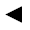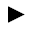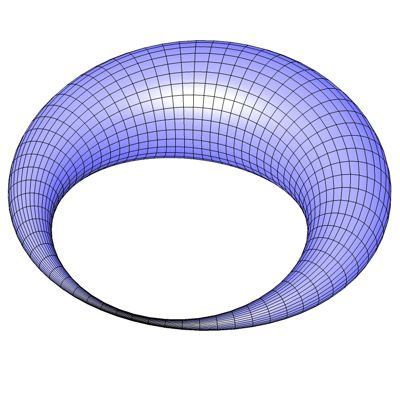[back]

## 3.55. Antisymmetric Torus

[next]The antisymmetric torus [ 16 ] is represented by the following equations.

 x = (R + r cos(v) (a + sin(u))) cos(u) 3-184 y = (R + r cos(v) (a + sin(u))) sin(u) 3-185 z = r sin(v) (a + sin(u)) 3-186

The constants R, r and a determine the appearance of the figure.

To represent the area, the two parameters u and v can assume the following values ​​(domain of definition), for example.

 u is an element from the set of numbers [0, 2 pi] v is an element of the number set [0, 2 pi]

Since the antisymmetric torus is a closed figure, the definition range must be adhered to exactly, it cannot be changed with the plugin.
The plugin creates an optimized mesh without duplicate points and unconnected polygons.Fig. 59

Die Figur kann auf der nächsten Seite mit einem Java-Applet von allen Seiten betrachtet und gedreht werden.